# 小学期间必须让孩子熟记的数学计算公式小学阶段的学生通常在学习上存在着总结归纳能力欠缺等问题，为了很好地帮助孩子系统地掌握小学阶段的数学知识，特意为孩子们整理和总结了小学数学一年级到六年级所有的计算公式。建议收藏起来，进行熟记哦!

小学数学图形计算公式

平面图形的周长

1.长方形的周长=（长+宽）×2，C=（a+b）×2

2.正方形的周长=边长×4，C=4a

3.直径=半径×2，d=2r；半径=直径÷2，r=d÷2

4.圆的周长=圆周率×直径=圆周率×半径×2，c=πd=2πr

平面图形的面积

1.长方形的面积=长×宽，S=ab

2.正方形的面积=边长×边长，S=a×a= a2

3.三角形的面积=底×高÷2，S=ah÷2

4.平行四边形的面积=底×高，S=ah

5.梯形的面积=（上底+下底）×高÷2，S=（a+b）h÷2

6.圆的面积=圆周率×半径×半径，S=πr2

7.长方体的表面积=（长×宽+长×高+宽×高）×2=（ab+ah+bh）×2

8.正方体的表面积=棱长×棱长×6，S=6 a2

9.圆柱的侧面积=底面圆的周长×高，S=ch

10.圆柱的表面积=上下底面面积+侧面积，S=2πr2 +2πrh

立体图形的体积

1.长方体的体积 =长×宽×高，V =abh

2.正方体的体积=棱长×棱长×棱长，V=a×a×a= a3

3.圆柱的体积=底面积×高，V=Sh，V=πr2h

4.圆锥的体积=底面积×高÷3，V=Sh÷3=πr2h÷3

具体情景问题

和、差、倍问题

（和+差）÷2=大数，（和-差）÷2=小数

和÷（倍数+1）=小数，小数×倍数=大数（或者 和-小数=大数）

差÷（倍数-1）=小数，小数×倍数=大数（或 小数+差=大数）

植树问题

（1 ）非封闭线路上的植树问题主要可分为以下三种情形：

a.如果在非封闭线路的两端都要植树，那么：

株数=段数+1=全长÷株距+1

全长=株距×（株数-1）

株距=全长÷（株数-1）

b.如果在非封闭线路的一端要植树，另一端不要植树，那么：

株数=段数=全长÷株距

全长=株距×株数

株距=全长÷株数

c.如果在非封闭线路的两端都不要植树，那么：

株数=段数-1=全长÷株距-1

全长=株距×（株数+1）

株距=全长÷（株数+1）您还可以通过手机、平板电脑等移动设备访问济南奥数网，升学路上有我们相伴。>>[点击查看]

•欢迎扫描二维码
关注奥数网微信
ID：aoshu_2003

•欢迎扫描二维码
关注中考网微信
ID：zhongkao_com

### 教育导航

1.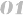北京站 上海站 广州站 深圳站
2.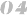天津站 武汉站 成都站 石家庄站
3.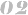南京站 杭州站 济南站 苏州站
4.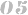郑州站 沈阳站 太原站 重庆站
5.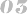长沙站 合肥站 宁波站 青岛站